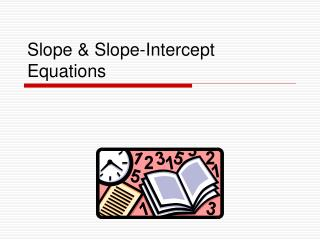DownloadDownload PresentationSlope & Slope-Intercept Equations

# Slope & Slope-Intercept Equations

Download Presentation## Slope & Slope-Intercept Equations

- - - - - - - - - - - - - - - - - - - - - - - - - - - E N D - - - - - - - - - - - - - - - - - - - - - - - - - - -
##### Presentation Transcript

1. Slope & Slope-Intercept Equations

2. IN LAYMAN’S TERMS: Slope is the measure of the steepness of a line!

3. HOW IT’S FIGURED: VERTICAL CHANGE ___________________ STEEPNESS = HORIZONTAL CHANGE **OR** Change in Y-axis ___________________ SLOPE (m) = Change in X-axis

4. Steps • Determine Point 1 & 2 • Label x1, y1, x2, y2 • Substitute in the formula:

5. WORK TIME! Find the slope of a line that contains points A(-2, 5) B(4, -5)

6. HERE’S HOW YOU SOLVE IT: Change in Y-axis ___________________ SLOPE (m) = Change in X-axis 5 – (-5) ___________________ m of line AB = -2 - 4 10 ___________________ = -6 5 ___________ - = 3

7. NOW TRY THESE ON YOUR OWN Find the slope of a line that contains each pair of points: • R(9, -2) S(3, -5) • M(7, -4) N(9, 4)

8. True or False?? ALL HORIZONTAL LINES HAVE THE SAME SLOPE

9. THE ANSWER! #1: All horizontal lines have the same slope. TRUE

10. TWO LINES MAY HAVE THE SAME SLOPE

11. THE ANSWER! #2: Two lines may have the same slope. TRUE

12. A LINE WITH A SLOPE OF 1 PASSES THROUGH THE ORIGIN

13. THE ANSWER! #3: A line with a slope of 1 passes through the origin. FALSE

14. Use the Slope to Graph a Line • You will need an ordered pair and the slope • Graph the line that passes through (1,4) and has a slope .